# Year 2 Maths Printable Worksheets

Use our colourful year 2 maths worksheets to help your child master new maths skills! Our maths worksheets cover all the core objectives including problem-solving, shape and space, mental maths, number bonds, and measuring and recording results using tables. We also cover topics such as money, fractions, greater than/less than, ordering and comparing, and more.

Filters

### Colouring shapes to show the fraction

This worksheet asks students to colour the shapes to show the fraction.

Age: 6 - 7
1
2
3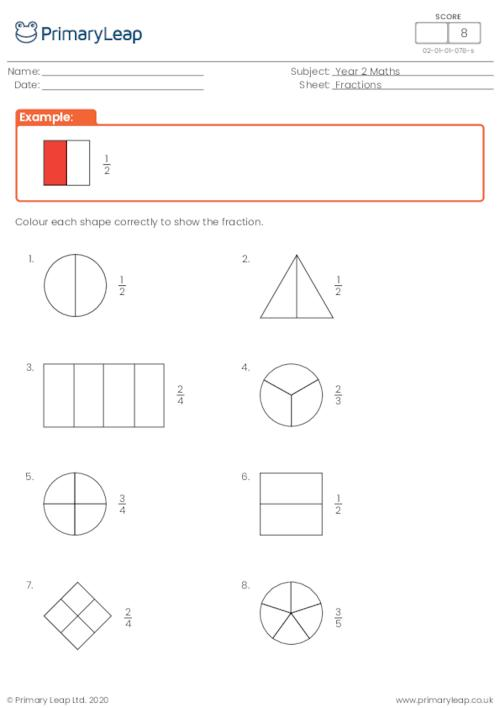### Dividing shapes into two equal parts

This worksheet asks students to divide each shape into two equal parts by drawing a line.

Age: 6 - 7
1
2
3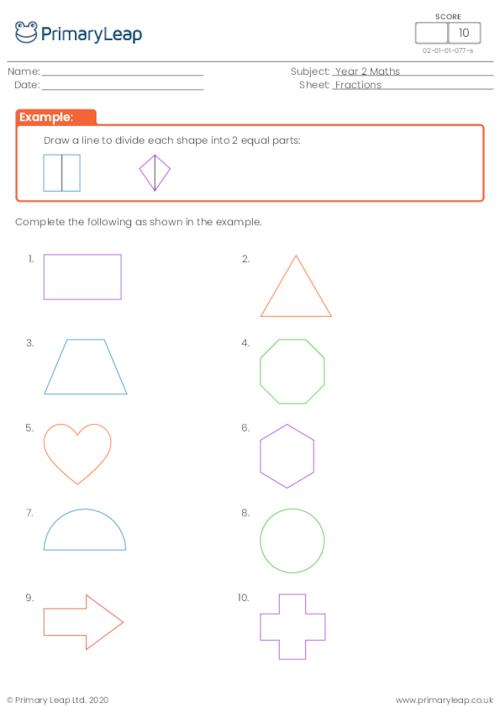### Matching fractions to words

This worksheet asks students to draw a line to match the fractions to the words. e.g. one fifth - 1/5

Age: 6 - 7
1
2
3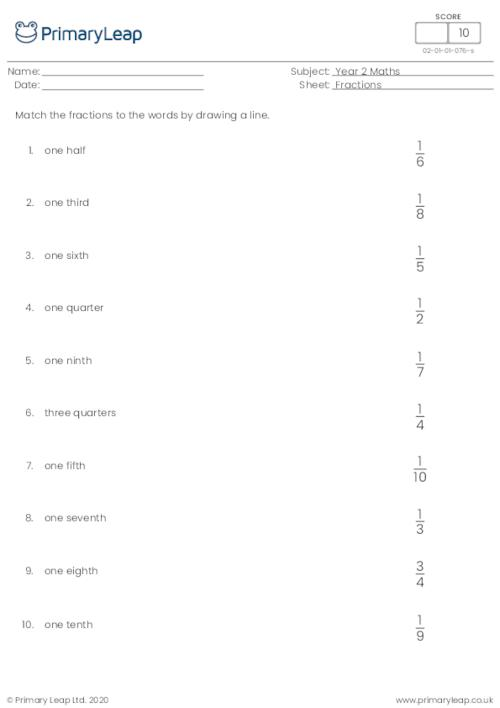### Bonds of 10 and 20

A KS1 maths worksheet on bonds of 10 and 20.

Age: 6 - 7
1
2
3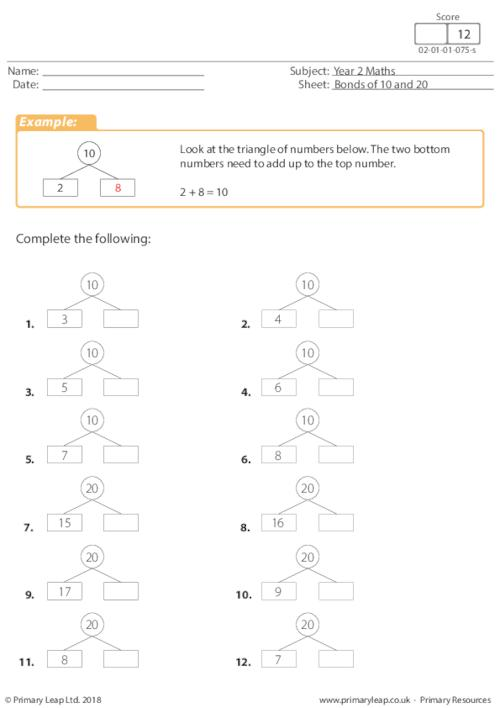### Sharing by 3

Year 2 Maths - This maths worksheet teaches children to share by 3. They must circle the apples and oranges into groups of 3 and answer the division questions.

Age: 6 - 7
1
2
3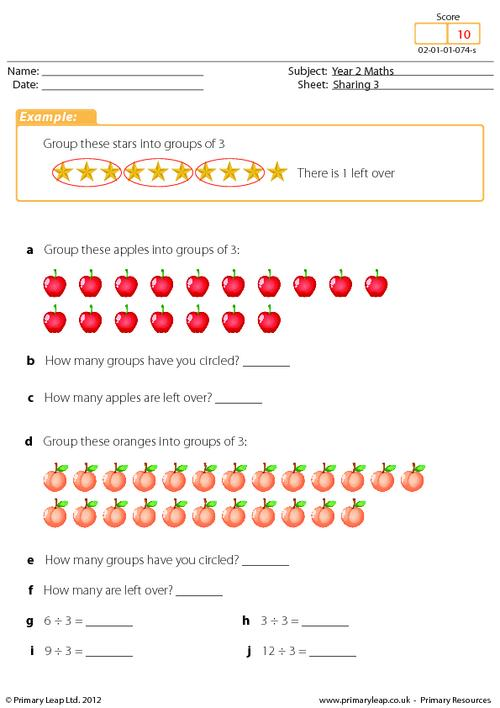### Sharing by 5

Year 2 Maths - This maths worksheet teaches children to share by 5. They must circle the apples and pears into groups of 5 and answer the division questions.

Age: 6 - 7
1
2
3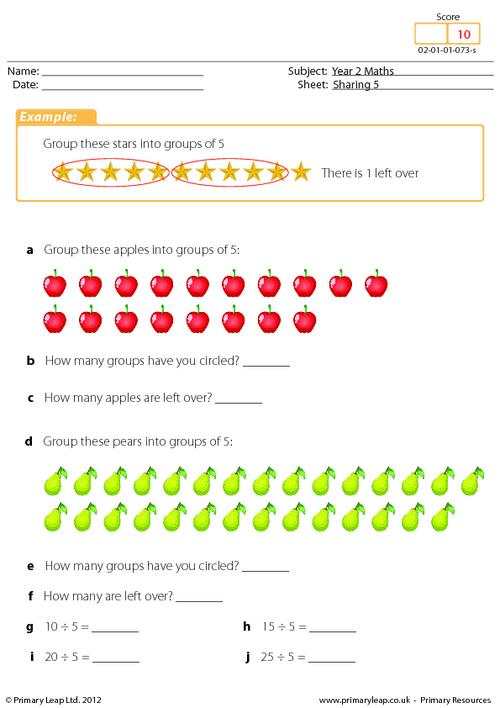Year 2 Maths - Students must colour the pairs of balloons the same colour so that the answer equals 20.

Age: 6 - 7
1
2
3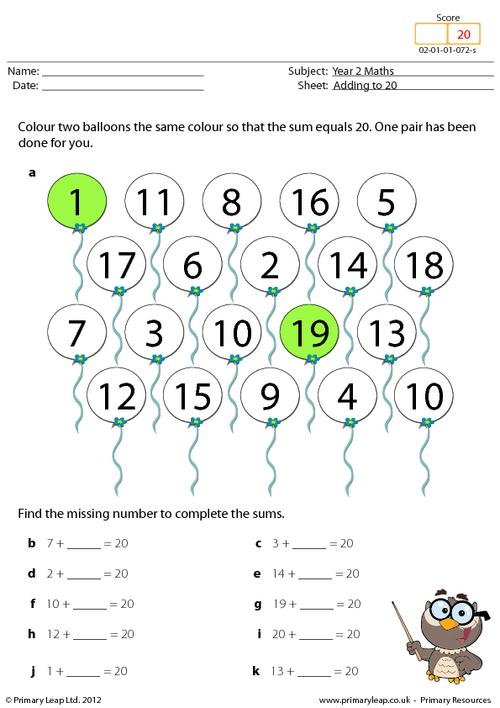### Using the correct numbers

Year 2 Maths - Students must use the correct numbers to complete the questions. They are allowed to use only the numbers given on the worksheet.

Age: 6 - 7
1
2
3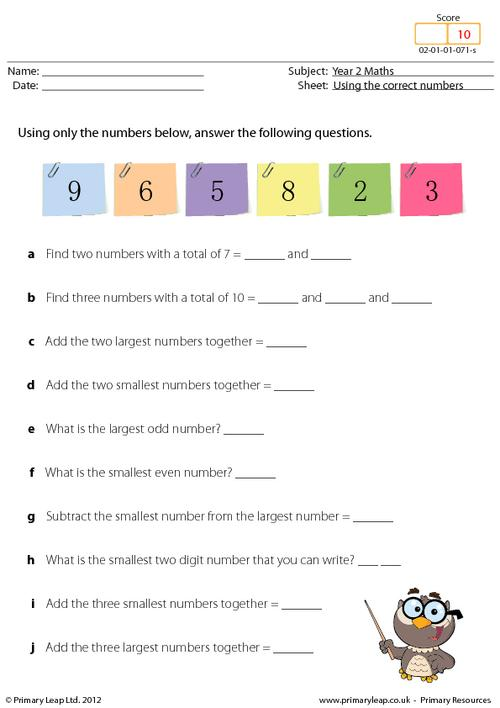### Writing numbers in words and digits

Year 2 Maths - This worksheet teaches children to write numbers in words and digits.

Age: 6 - 7
1
2
3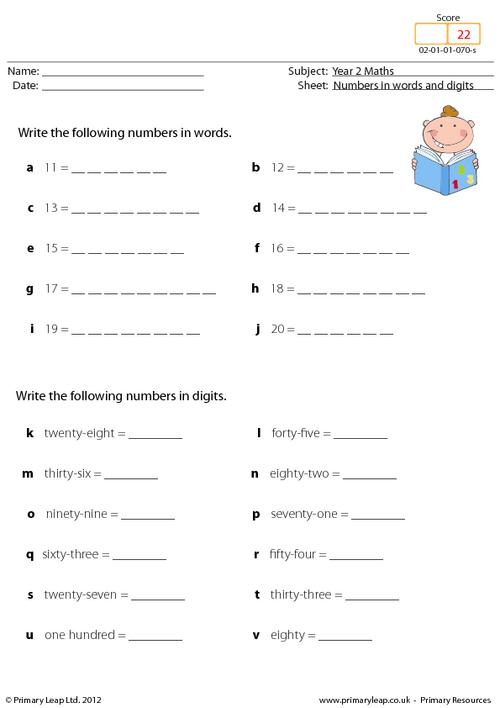### Multiplication Square Mixed

Year 2 maths - Multiplication square

Age: 6 - 7
1
2
3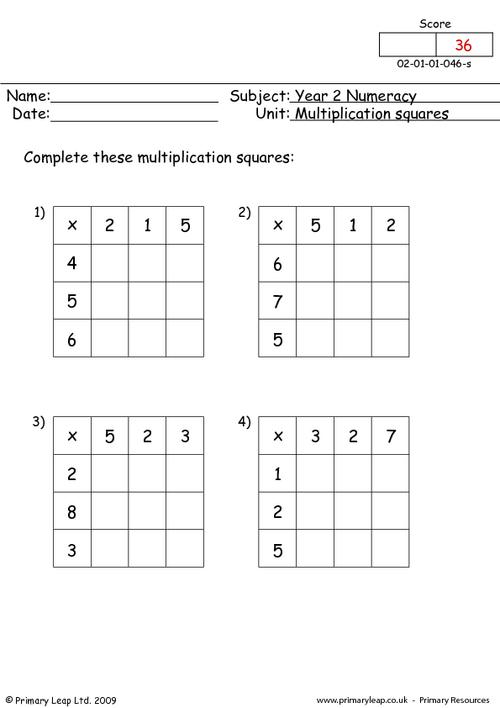Year 2 maths - A year 2 numeracy worksheet on number bonds of 100 and 1000. Number bonds greatly help your child with addition skills throughout their school lives.

Age: 6 - 7
1
2
3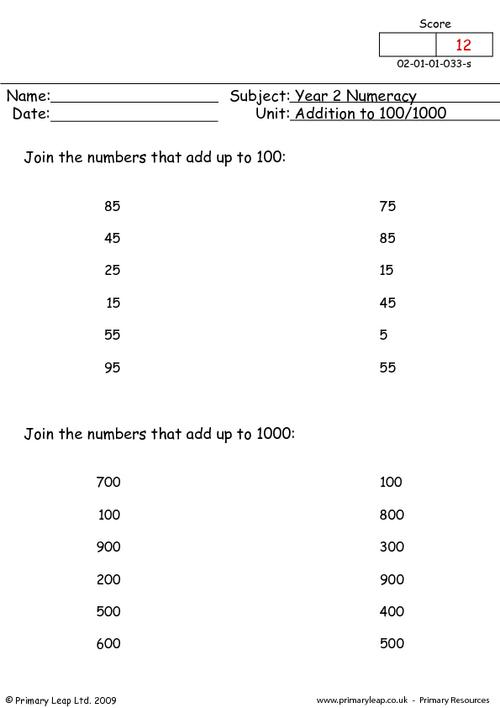### Rounding To The Nearest 10

Year 2 maths - Rounding worksheet.

Age: 6 - 7
1
2
3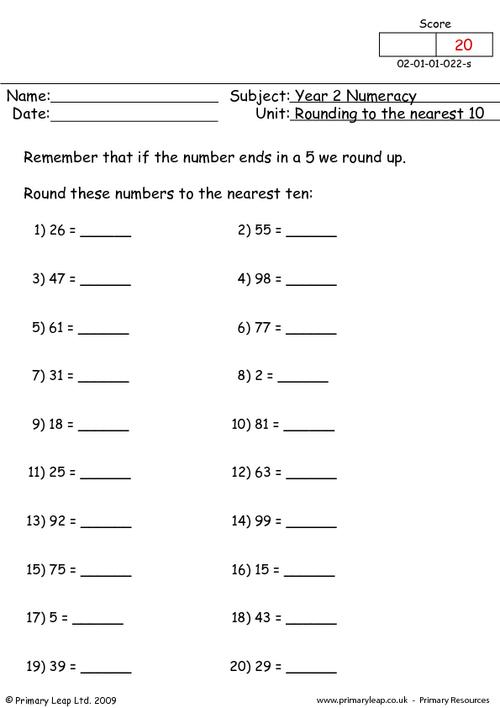### Sequencing 1

Year 2 maths - A year 2 numeracy worksheet on sequencing. Children will need to look at the rule for each sequence and then fill in the missing numbers in each sequence.

Age: 6 - 7
1
2
3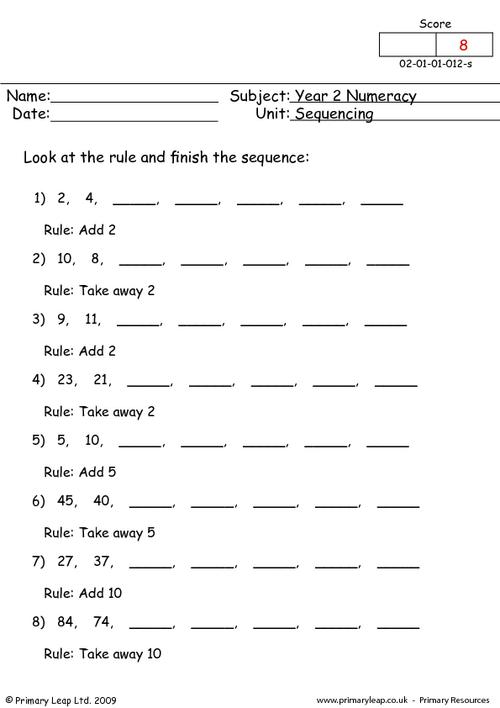### Ordering Numbers (4)

Year 2 maths - Ordering worksheet. Children are asked questions to order the days of the week and the seasons of the year.

Age: 6 - 7
1
2
3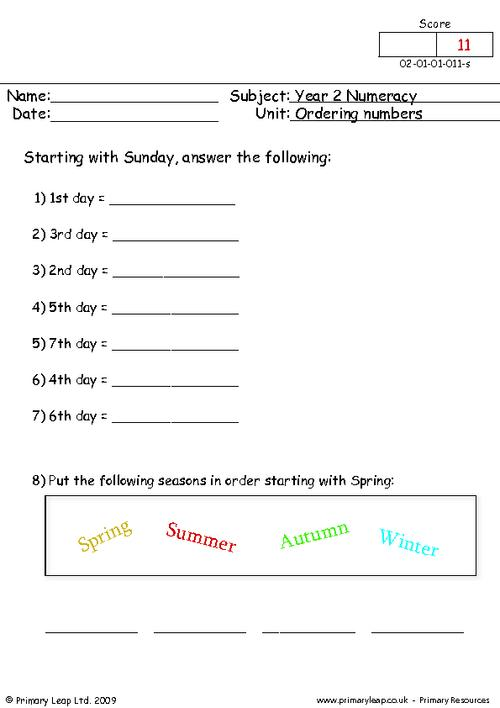### Ordering Numbers (3)

Year 2 maths - Ordering numbers worksheet. Children are asked to put the numbers in order starting with the smallest.

Age: 6 - 7
1
2
3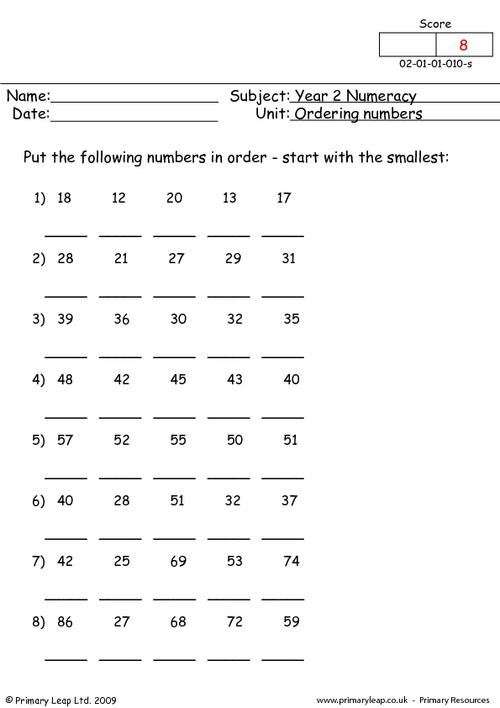### Odd & Even (3)

Year 2 maths - Odd and even worksheet. This worksheet asks children to colour the apples red if the answer is even and green if the answer is odd.

Age: 6 - 7
1
2
3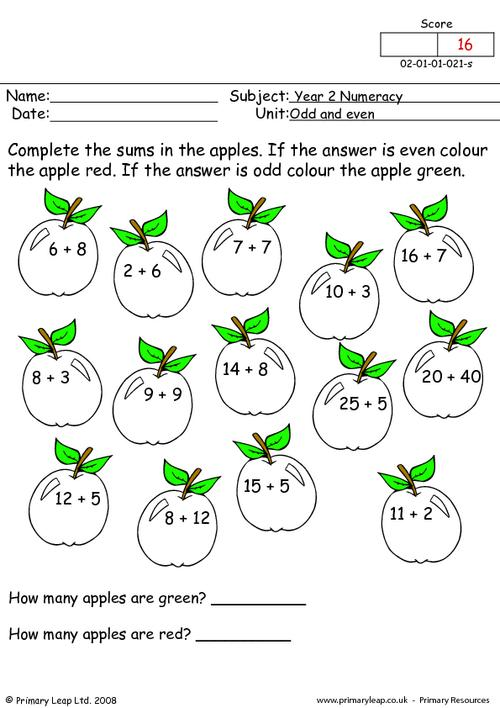### Writing numbers 1

Year 2 maths - A year 2 numeracy worksheet on recognising numbers and figures. The child will need to join up the words and figures that are the same.

Age: 6 - 7
1
2
3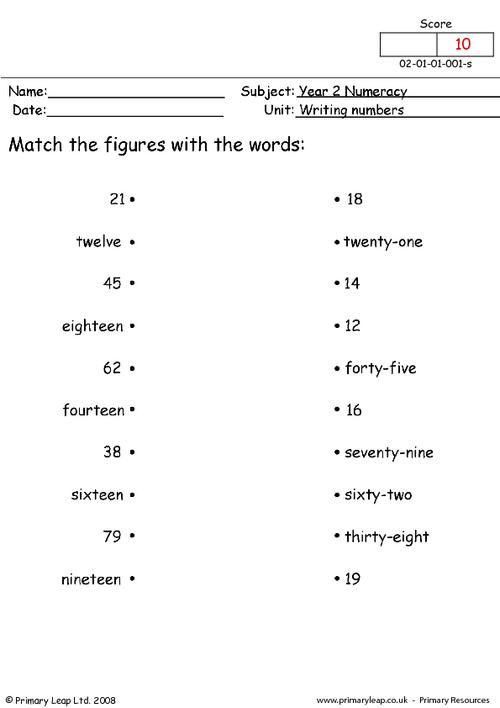### Fractions review

Year 2 maths - Revision worksheet on fractions. This worksheet is a short test of fractions at year 2 level covering recognising fractions and fractions of an amount.

Age: 6 - 7
1
2
3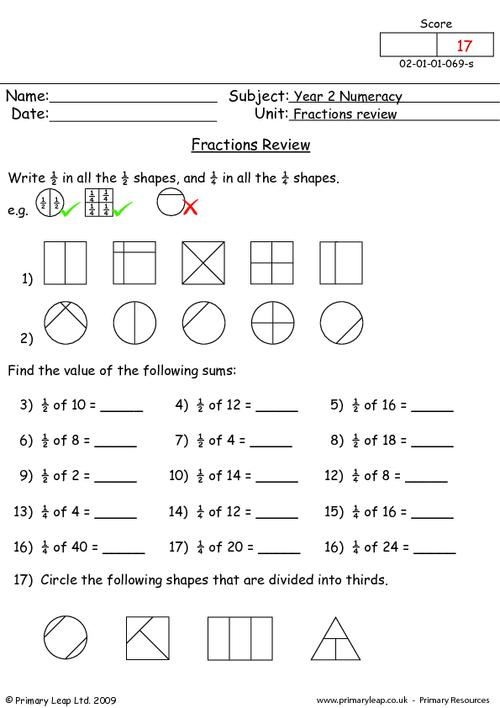### Division review

Year 2 maths - Revision worksheet including mixed questions on division.

Age: 6 - 7
1
2
3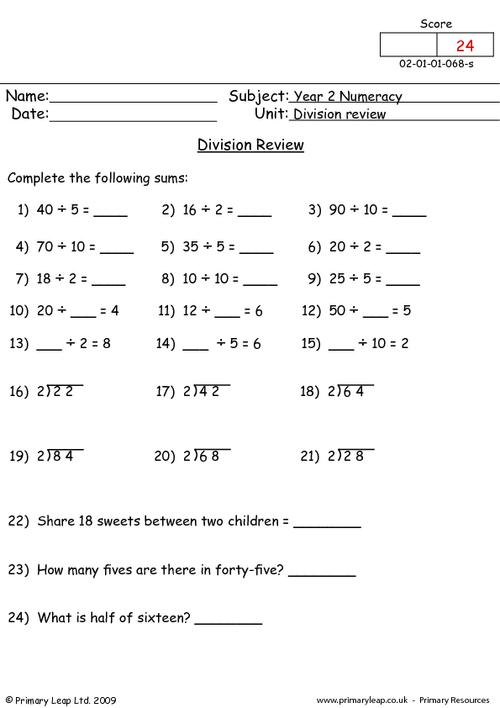### Multiplication review

Year 2 maths - Revision worksheet including horizontal, vertical and written questions on multiplication.

Age: 6 - 7
1
2
3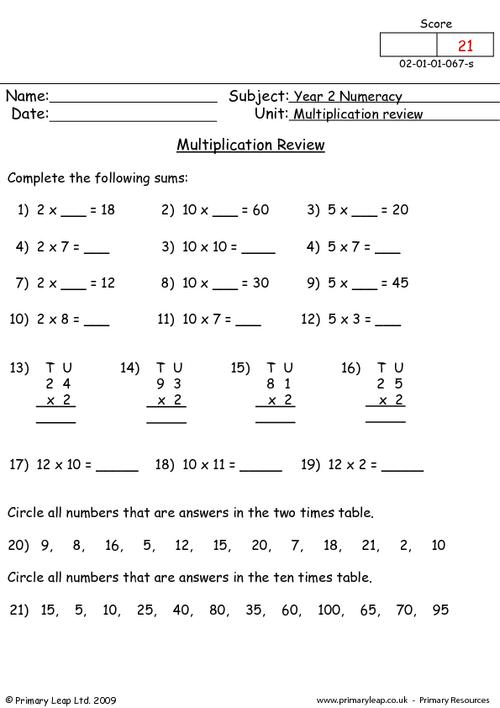### Subtraction review

Year 2 maths - Revision worksheet including horizontal, vertical and written questions on subtraction.

Age: 6 - 7
1
2
3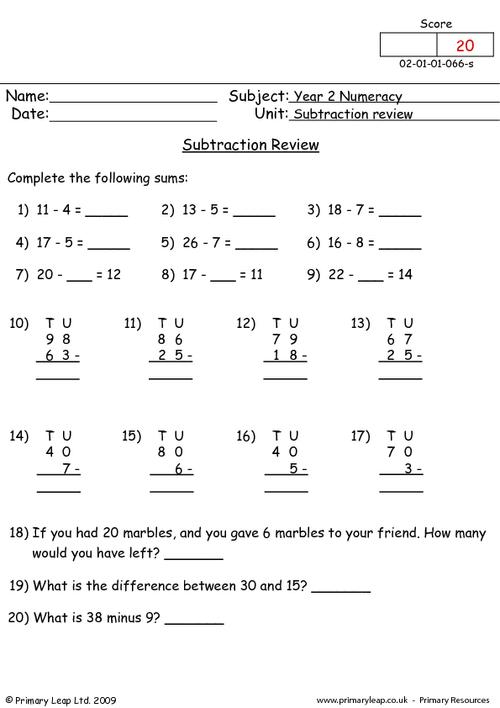Year 2 maths - Revision worksheet including both horizontal and vertical addition sums. When pupils can complete this worksheet they will fully understand this topic up to year 2 standard.

Age: 6 - 7
1
2
3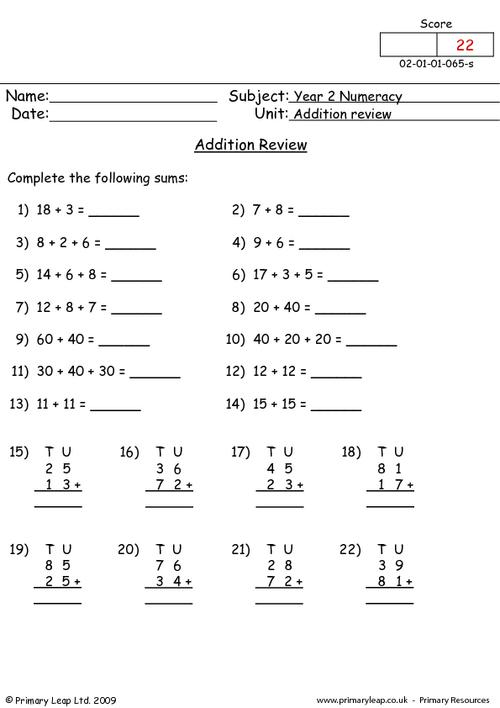### Ordering numbers review

Year 2 maths - Revision worksheet including questions on ordering numbers.

Age: 6 - 7
1
2
3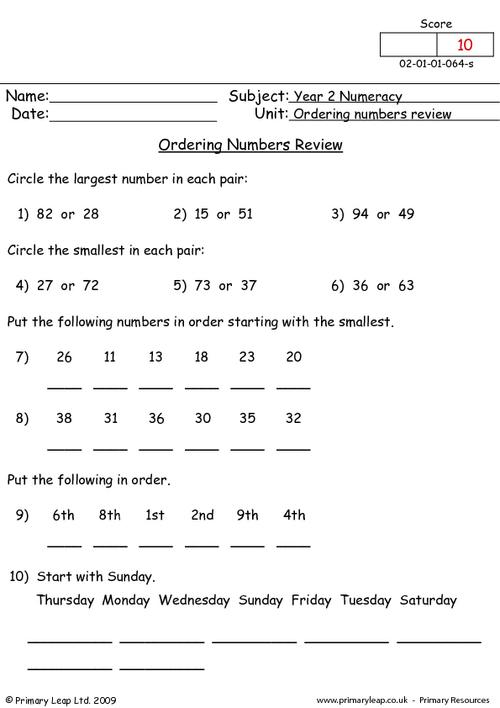### Odd and even review

Year 2 maths - Revision worksheet including questions on odd and even numbers.

Age: 6 - 7
1
2
3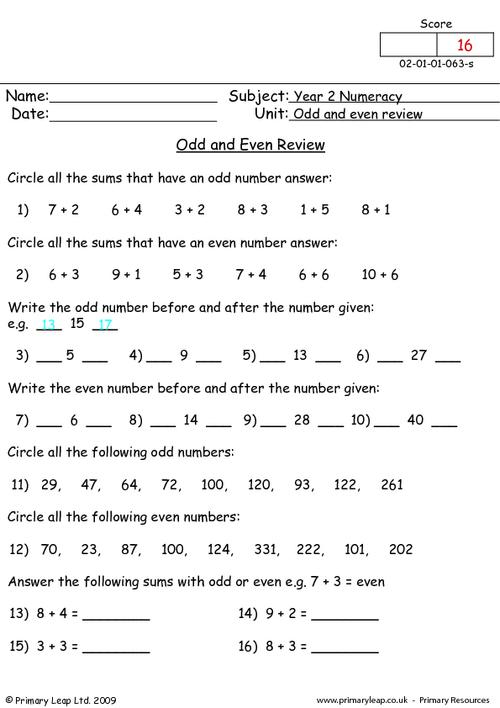### Tens Review

Year 2 maths - A revision worksheet for year 2 pupils on tens. Sequencing is also covered.

Age: 6 - 7
1
2
3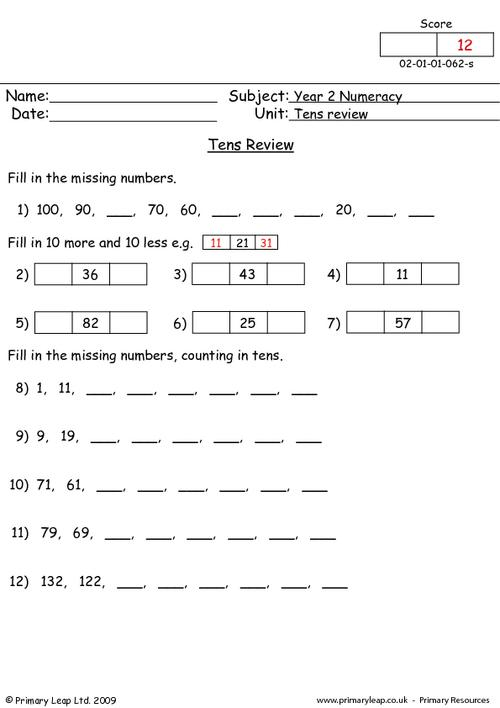### Writing numbers and place value review

Year 2 maths - Revision worksheet. This is a useful resource for revision of writing numbers and place value.

Age: 6 - 7
1
2
3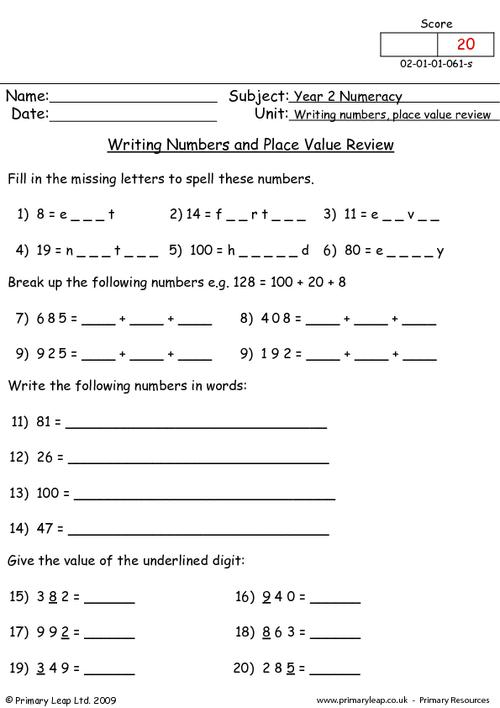### Times tables chart

Year 2 maths - Multiplication tables. These tables include the 1, 2, 5 and 10 times table.

Age: 6 - 7
1
2
3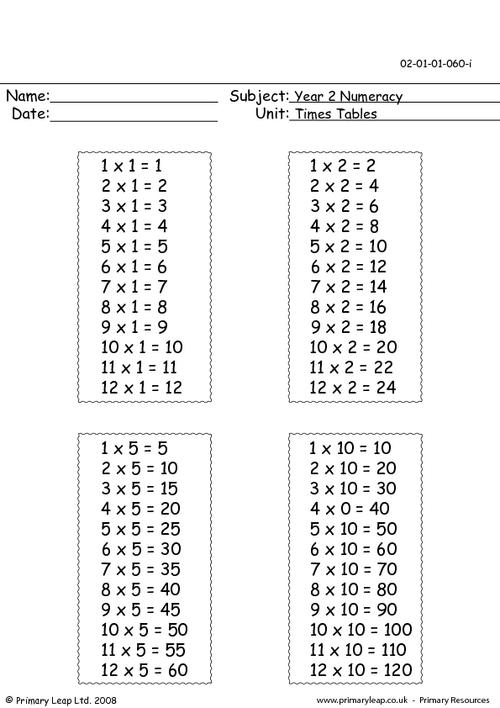### Multiplication square

Year 2 maths - Multiplication square. This multiplication square can be used when learning your times tables. 1 - 10 times table.

Age: 6 - 7
1
2
3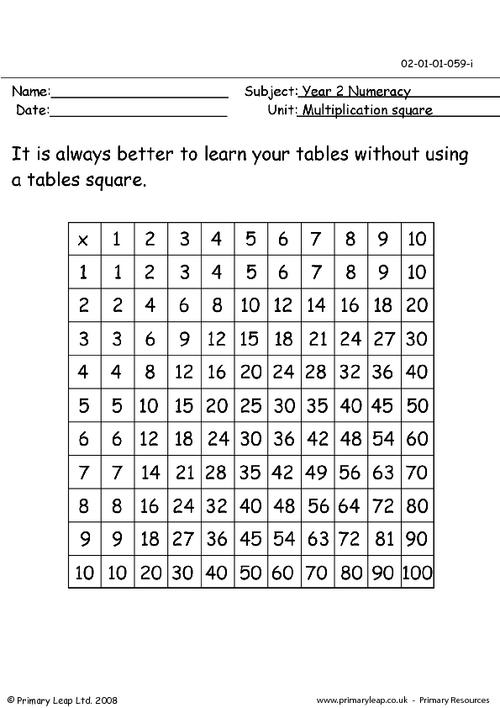### Fractions (4)

Year 2 maths - Fractions. This worksheet asks children to give half, quarter and a third of a given number.

Age: 6 - 7
1
2
3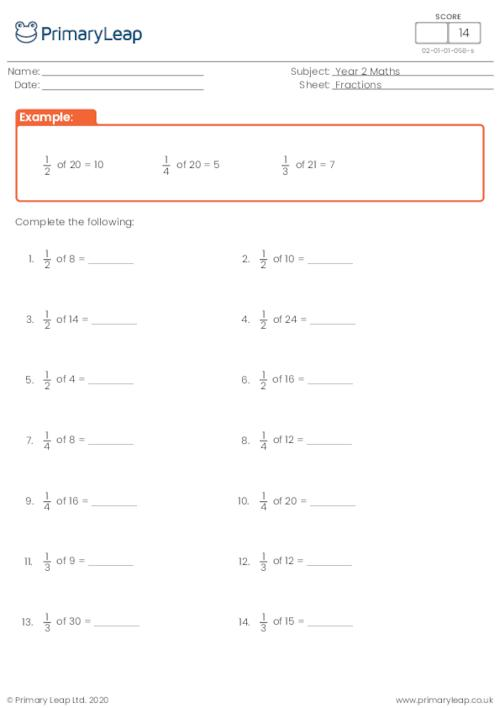### Fractions (3)

Year 2 maths - Fraction of shapes worksheet. This worksheet asks children to tick the shapes which are quarters and cross the shapes that are not.

Age: 6 - 7
1
2
3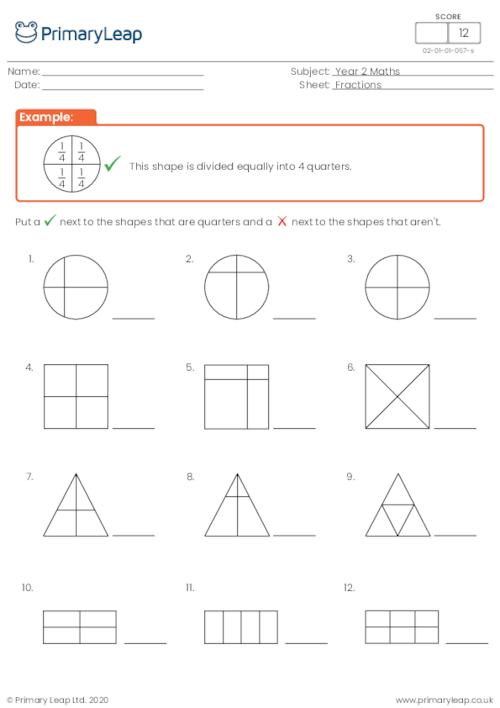### Fractions (2)

Year 2 maths - Fraction of shapes worksheet. This worksheet asks children to tick the shapes which are halves and cross the shapes that are not.

Age: 6 - 7
1
2
3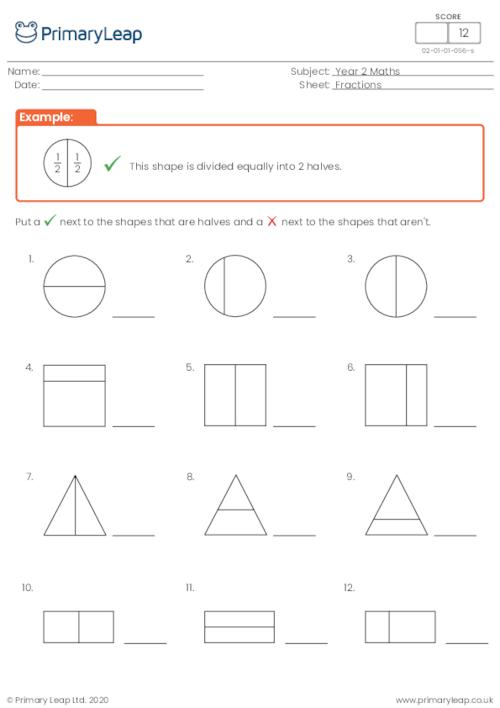### Fractions of shapes

Year 2 maths - Fraction of shapes worksheet. Children are asked to write the fraction of each shape.

Age: 6 - 7
1
2
3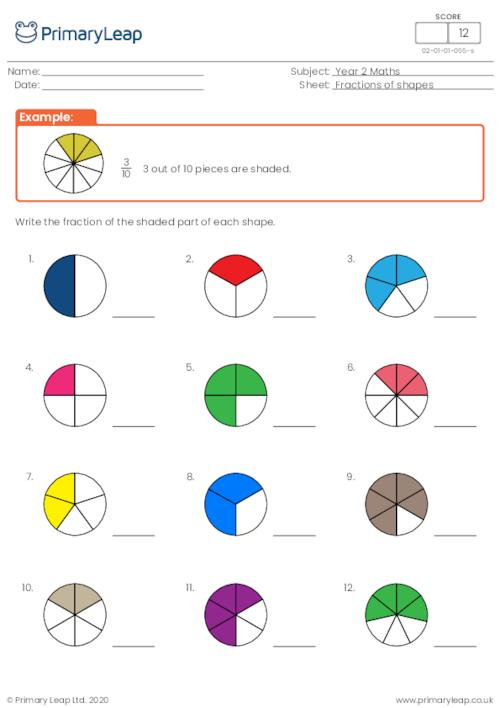### Which operation?

Year 2 maths - Which operation? This worksheet asks children to choose the correct sign to complete the sums.

Age: 6 - 7
1
2
3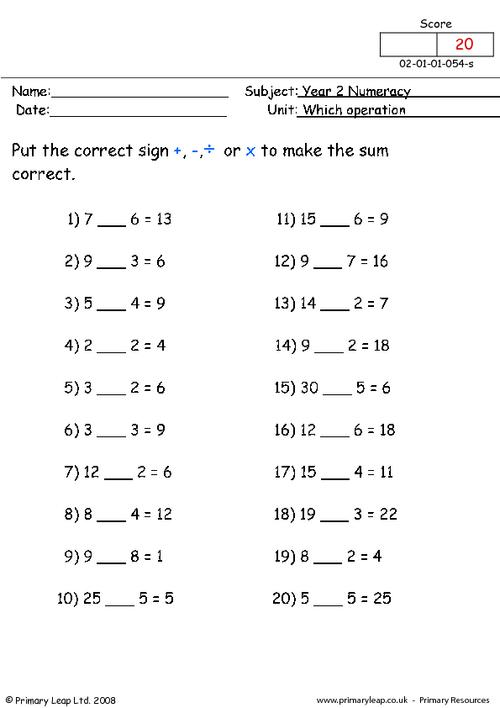### Division problems

Year 2 maths - Division worksheet. This worksheet includes 10 written questions on division.

Age: 6 - 7
1
2
3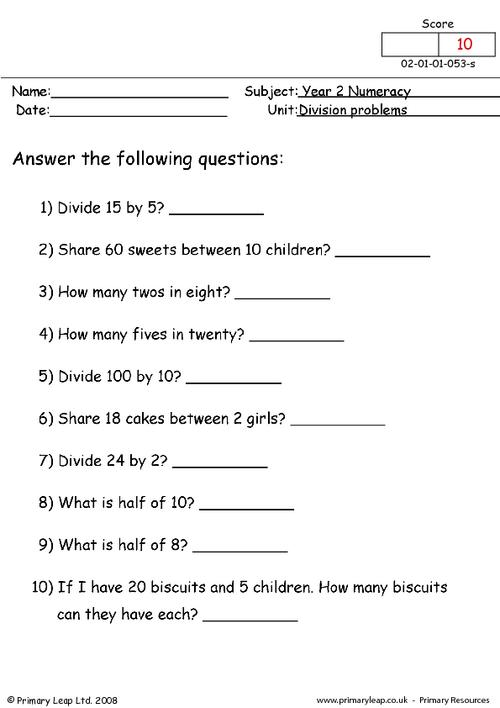### Divide by 5

Year 2 maths - A year 2 maths worksheet on dividing by 5. This primary resource includes straight forward sums and a section on missing numbers.

Age: 6 - 7
1
2
3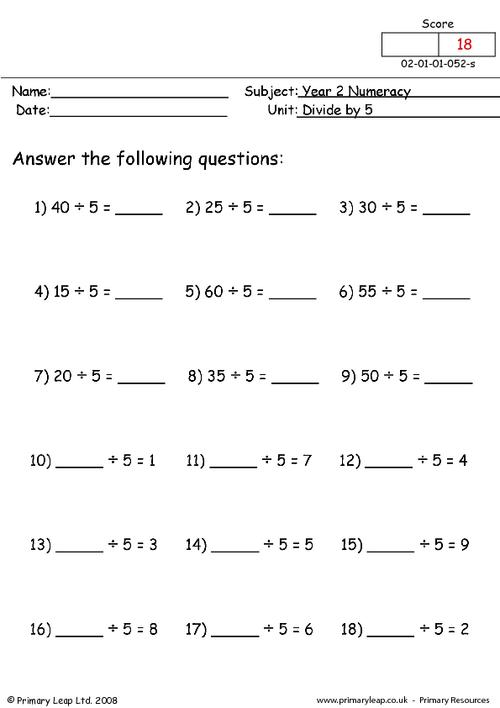### Divide by 10

Year 2 maths - Division worksheet. This worksheet asks children to divide by 10 and fill in the missing numbers to complete the sums.

Age: 6 - 7
1
2
3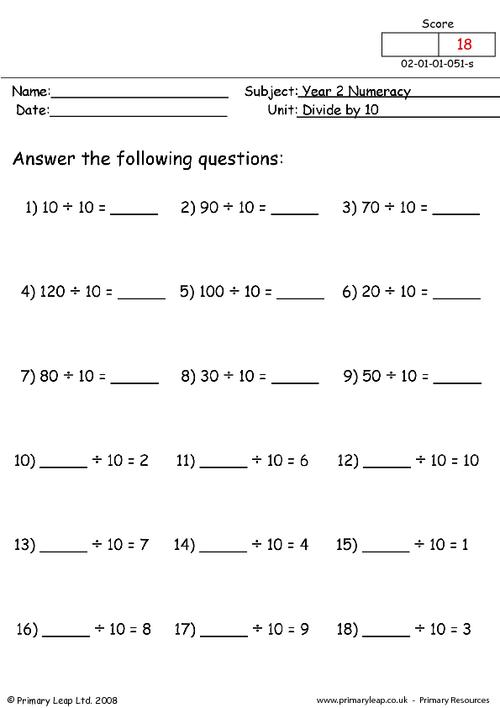### Divide by 2

Year 2 maths - Division worksheet. This worksheet asks children to divide by 2.

Age: 6 - 7
1
2
3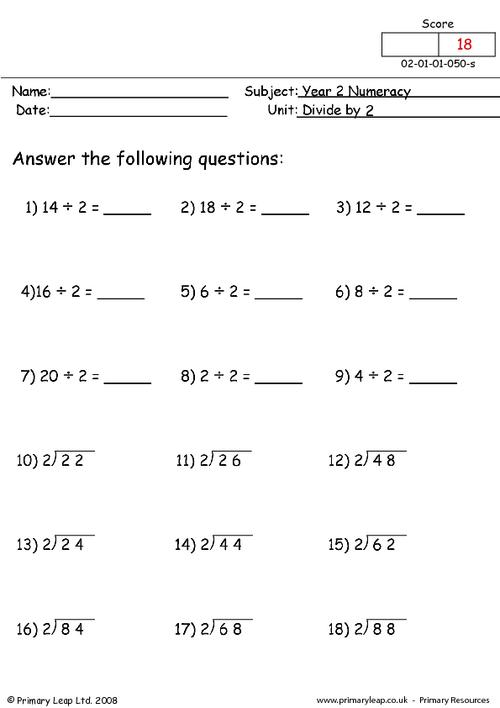### Money (2)

Year 2 maths - This worksheet includes 18 questions on money subtraction. All amounts are under 1 pound.

Age: 6 - 7
1
2
3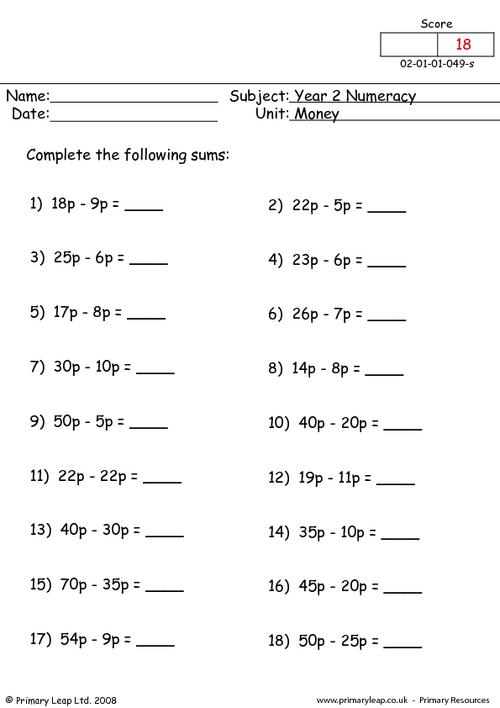### Money (1)

Year 2 maths - A year 2 numeracy worksheet on money. The addition sums on this sheet have two amounts of under 1 pound to add together.

Age: 6 - 7
1
2
3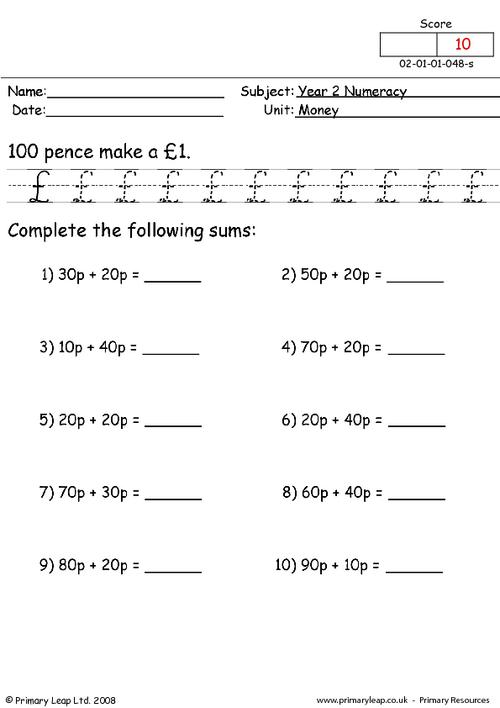### Multiplication problems

Year 2 maths - This worksheet includes written multiplication questions.

Age: 6 - 7
1
2
3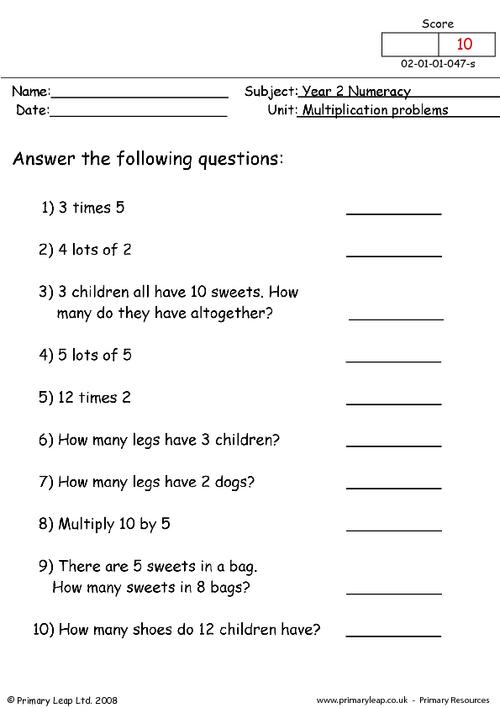### Multiplication

Year 2 maths - This multiplication worksheet includes questions on the 2, 5 and 10 times table.

Age: 6 - 7
1
2
3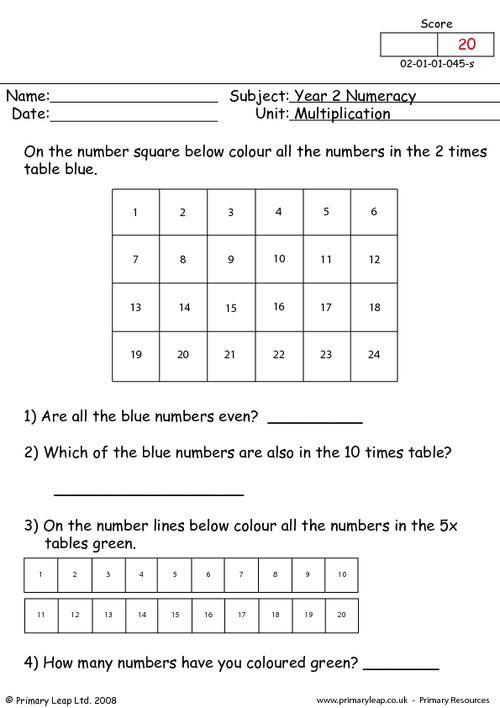### 5 x tables

Year 2 maths - Multiplication worksheet. Learning multiplication tables is very important and will help children with their maths skills.

Age: 6 - 7
1
2
3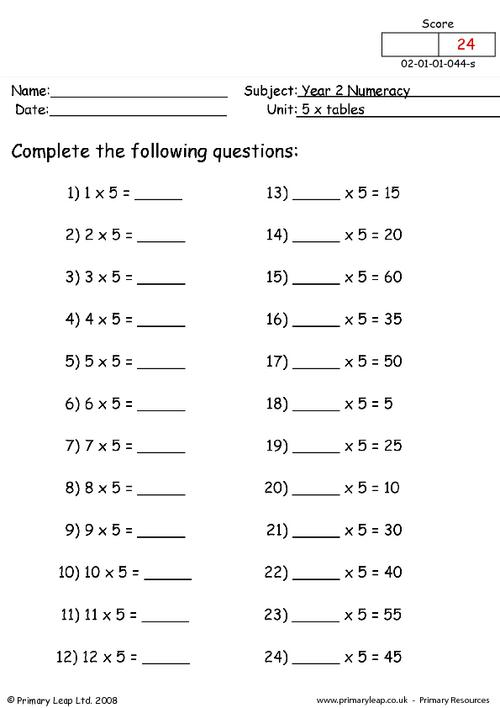### 10 x tables

Year 2 maths - 10 time table. This multiplication worksheet includes 18 sums on the 10 times table.

Age: 6 - 7
1
2
3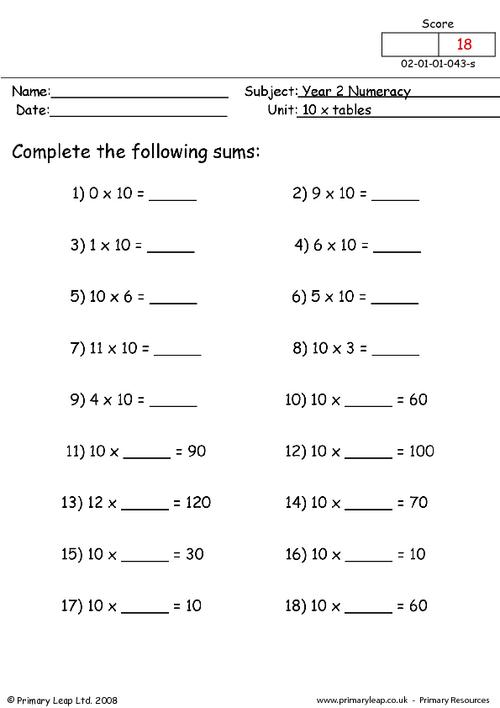### Multiplying by 2

Year 2 maths - This worksheet includes both horizontal and vertical multiplication sums.

Age: 6 - 7
1
2
3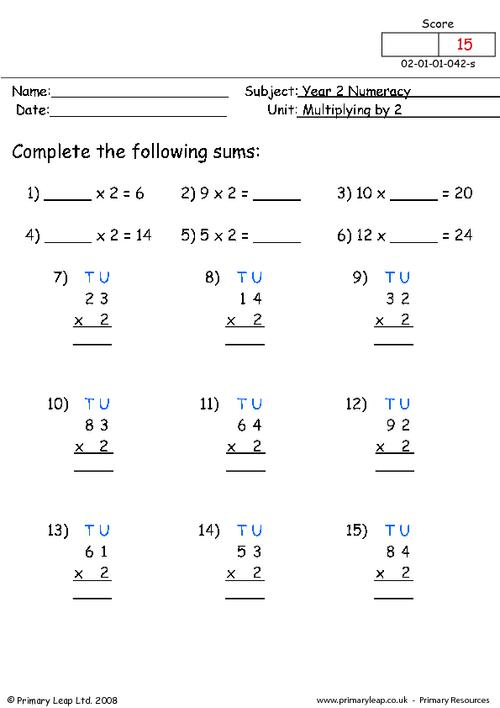### Multiply by 2

Year 2 maths - Multiplication worksheet. This printable introduces multiplication by 2.

Age: 6 - 7
1
2
3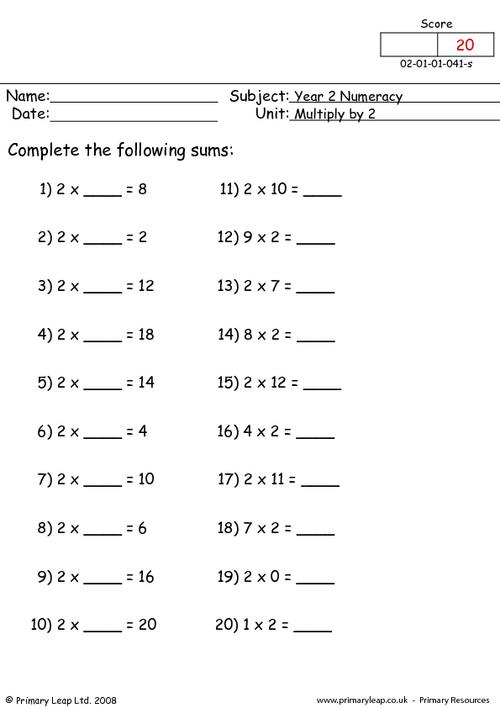### Subtraction vertical (2)

Year 2 maths - Vertical subtraction worksheet with carrying.

Age: 6 - 7
1
2
3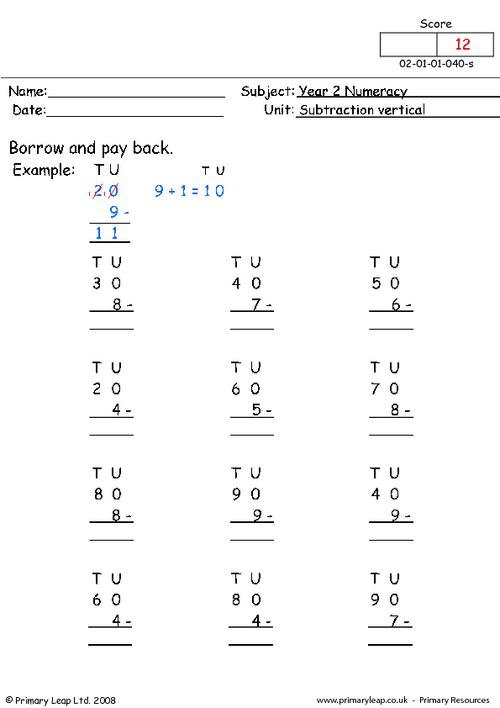### Subtraction vertical (1)

Year 2 maths - Vertical subtraction worksheet with no carrying.

Age: 6 - 7
1
2
3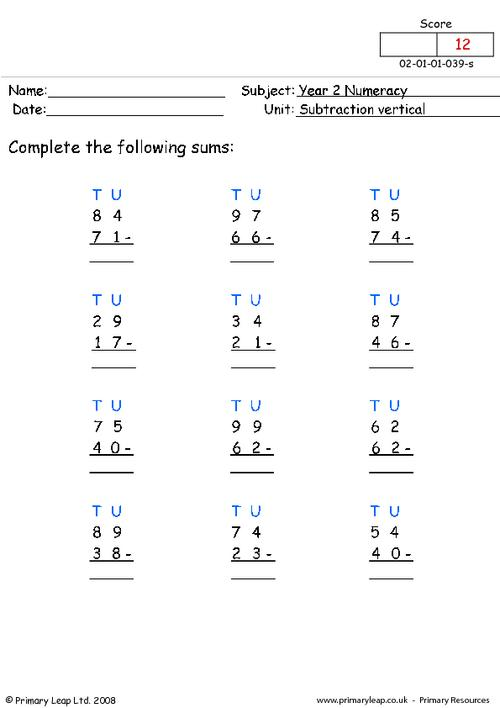### Subtraction

Year 2 maths - Subtraction worksheet. There are 20 subtraction sums for pupils to complete.

Age: 6 - 7
1
2
3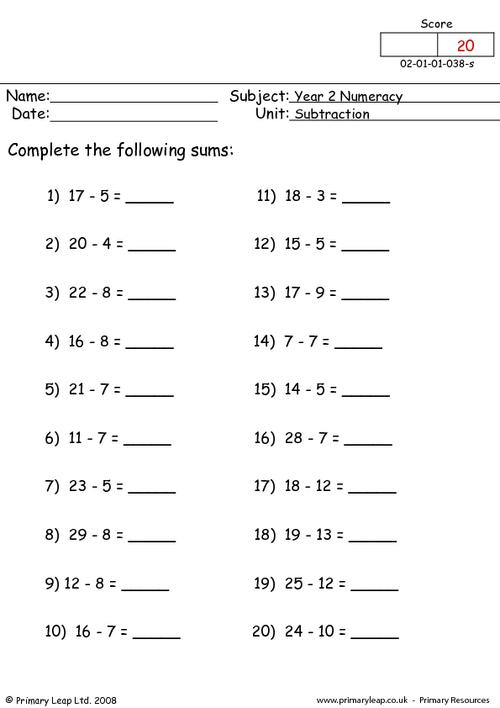of 2Help your child find the joy of learning year 2 maths with our engaging maths activities. We have hundreds of year 2 maths worksheets to boost your child's confidence. Our maths worksheets will help your child learn how to count in steps of 2, 3, 5 and 10, learn number bonds to 20, add and subtract one-digit and two-digit numbers, find fractions of shapes, and more.Learn

One of the best things about printable worksheets is that they make learning fun and easy. Explore our educational resources based on Year 2 Maths and keep your children learning through these fun and engaging exercises.Progress

Our Year 2 Maths printable worksheets are designed for progressive learning. Our engaging content enables a child to learn at their own speed and gain confidence quickly. When we help a child believe in themselves, we empower them for life.Improve

Printable worksheets are a great way to enhance creativity and improve a child’s knowledge. Help your child learn more about Year 2 Maths with this great selection of worksheets designed to help children succeed.